##### Organic Chemistry I For DummiesAny chiral center can have two possible configurations, and these configurations are designated either R or S by convention (the letters R and S come from the Latin words for right and left, rectus and sinister). If a molecule has a chiral center that is designated R, the chiral center will be S in the molecule's enantiomer.

You need to be able to assign whether a chiral center is R or S. To do so, you need to follow three steps:

1. Number each of the substituents on the chiral center carbon using the Cahn–Ingold–Prelog system.

According to the Cahn–Ingold–Prelog prioritizing scheme, the highest priority goes to the substituent whose first atom has the highest atomic number. (For example, Br would be higher priority than Cl, because Br has a larger atomic number.) If the first atoms on two substituents are the same, you keep going down the chain until you reach the first higher-priority atom and the tie is broken.

2. After you've assigned priorities to each of the substituents, rotate the molecule so that the number-four priority substituent is oriented in the back.

3. Draw a curve from the first-priority substituent through the second-priority substituent and then through the third.

If the curve goes clockwise, the chiral center is designated R; if the curve goes counterclockwise, the chiral center is designated S.

Here's an example: Try to determine the R/S stereochemistry of the chiral center in the molecule shown in the figure.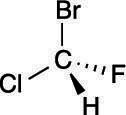A chiral molecule.

## Step 1: Prioritizing the substituents

The first step is to prioritize all the substituents from one to four. Bromine is the atom with the largest atomic number, so this substituent is given the highest priority; hydrogen has the smallest atomic number, so it's given the lowest priority. Chlorine gets the number-two priority because it has a higher atomic number than fluorine, which is given priority three. The priorities are shown here.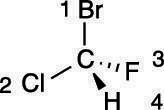Prioritizing the substituents in a chiral center.

Hydrogen will always be given the lowest (fourth) priority.

## Step 2: Putting the number-four substituent in the back

The next step is to rotate the molecule so that the number-four substituent is pointed toward the back, as shown here. For many people, this is the most difficult step because to rotate the molecule requires visualizing the molecule in three dimensions.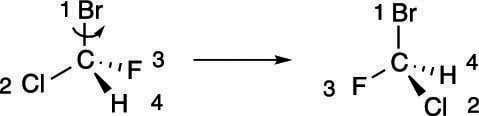Rotating the molecule to put the fourth priority in the back.

If you're not good at visualizing in three dimensions, you can use some tricks to put the number-four priority group in the back without having to mentally rotate the molecule in three-dimensional space.

First, swapping any two substituents changes the configuration — that is, if the chiral center was R before the swap, it becomes S after the swap (and vice versa). So, if you swap the number-four substituent with the substituent located in the back, as shown here, the configuration of the chiral center switches.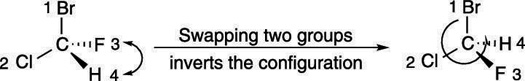Swapping two groups in a chiral center.

After you've made the switch to put the number-four priority substituent in the back, you could then go to Step 3 and determine the configuration, remembering that the actual configuration of that center is the opposite of the one determined.

Or you could keep going, swapping two more positions. Swapping the positions of the first two substituents inverts the configuration, but swapping the remaining two substituents after you perform this operation reverts the configuration back to the one you started with, as shown here.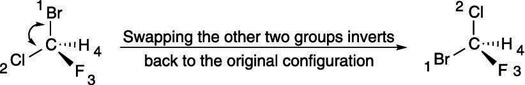Swapping two more groups in a chiral center.

So, if you first swap the number-four substituent with the group in the back, and then swap the remaining two substituents, you get two inversions of the configuration, which amounts to a net retention of the configuration. (For example, if the chiral center starts R, and you invert two substituents, it becomes S; if you invert two more substituents, the configuration goes back to R.) Doing this double swap is an easy way of getting the number-four priority substituent into the back without doing mental rotations of the atoms.

Swapping the positions of two substituents inverts the configuration.

## Step 3: Drawing the curve

Now that the number-four priority substituent is in the back, you draw a curve from the first-priority substituent through the second-priority substituent, to the third-priority substituent. In this case, the curve goes clockwise, so the molecule has R stereochemistry, as shown here.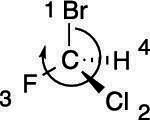The R stereochemistry.